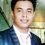# Complicated Set Theory Problems: Sequence of Sets

Let $$A_1, A_2, \ldots , A_n$$ be sets such that $$X = \bigcup_{i=1}^n A_i$$. Prove that there exists a sequence of sets $$B_1, B_2, \ldots , B_n$$ such that

a) $B_i \subseteq A_i$ for each $i=1,2,\ldots ,n$.

b) $B_i \cap B_j = \varnothing$ for $i\ne j$.

c) $X = \bigcup_{i=1}^n B_i$.

Can you give insights on how to solve this problem? Insights is enough for me.Note by Mharfe Micaroz
2 years, 7 months ago

This discussion board is a place to discuss our Daily Challenges and the math and science related to those challenges. Explanations are more than just a solution — they should explain the steps and thinking strategies that you used to obtain the solution. Comments should further the discussion of math and science.

When posting on Brilliant:

• Use the emojis to react to an explanation, whether you're congratulating a job well done , or just really confused .
• Ask specific questions about the challenge or the steps in somebody's explanation. Well-posed questions can add a lot to the discussion, but posting "I don't understand!" doesn't help anyone.
• Try to contribute something new to the discussion, whether it is an extension, generalization or other idea related to the challenge.

MarkdownAppears as
*italics* or _italics_ italics
**bold** or __bold__ bold
- bulleted- list
• bulleted
• list
1. numbered2. list
1. numbered
2. list
Note: you must add a full line of space before and after lists for them to show up correctly
paragraph 1paragraph 2

paragraph 1

paragraph 2

[example link](https://brilliant.org)example link
> This is a quote
This is a quote
    # I indented these lines
# 4 spaces, and now they show
# up as a code block.

print "hello world"
# I indented these lines
# 4 spaces, and now they show
# up as a code block.

print "hello world"
MathAppears as
Remember to wrap math in $$ ... $$ or $ ... $ to ensure proper formatting.
2 \times 3 $2 \times 3$
2^{34} $2^{34}$
a_{i-1} $a_{i-1}$
\frac{2}{3} $\frac{2}{3}$
\sqrt{2} $\sqrt{2}$
\sum_{i=1}^3 $\sum_{i=1}^3$
\sin \theta $\sin \theta$
\boxed{123} $\boxed{123}$

Sort by:

Just look at the "new" things added to $X$ by $A_i$. Call that $B_i$. For example $B_1$ is $A_1$, $B_2$ is $A_2 \setminus A_1$, etc. Can you continue from here?

- 2 years, 7 months ago

Nope. I cannot still comprehend.

- 2 years, 7 months ago

What to do next.

- 2 years, 7 months ago

$B_1$ is $A_1$. $B_2$ is $A_2 \setminus B_1$. $B_3$ is $A_3 \setminus B_2$. $B_4$ is $A_4 \setminus B_3$.

Now just verify that the claims given hold for these $B_i$'s

- 2 years, 7 months ago

Can you give me the first one? I will do the rest. I just need a guide. Thanks.

- 2 years, 7 months ago

Sorry, I made some typo. Define $B_1$ to be $A_1$. For $i > 1$, define $B_i$ to be $A_i \setminus (B_1 \cup B_2 \cdots \cup B_{i-1})$. The intution behind defining $B_i$ is simply to take all the elements which are new, i.e, the ones that you have not seen before.

• The first one is clear since each $B_i$ is defined to be $A_i \setminus B_{i-1}$. So, by definition of set difference, if something is in $B_i$, it must also be in $A_i$.
• For the second one, just notice that $B_i$ does not contain any element from $B_j$ for $i > j$. Thus, given two indices $i$ and $j$, it must always be the case that they are disjoint.
• If there is an element $x \in X$, then $x$ must be in some $A_i$. If $i_0$ is the least such value of $i$, then $x$ must be in $B_{i_0}$, and hence in $\bigcup_{i=1}^n B_i$. This shows that $X \subseteq \bigcup_{i=1}^n B_i$. Showing the other side is easy.

- 2 years, 7 months ago

Thank you so much. This is enough already.

- 2 years, 7 months ago

No problem

- 2 years, 7 months ago

Do you mean the following Sir?

That for (a) I will assume first that $x\in A_k$ and do all ways to prove that $x\in B_k$ given $B_i \subseteq A_i$ for each $i=1,2,\ldots ,n$?

For (b), I need to prove that $B_i$ is a disjoint set such that $A_{i} \setminus B_{i-1} \bigcap A_{j} \setminus B_{j-1}=\emptyset$ ?

Lastly, for (c), I need to prove that $B_i=B_k$ which implies that $A_{i} \setminus B_{i-1} = A_{j} \setminus B_{j-1}$?

- 2 years, 7 months ago